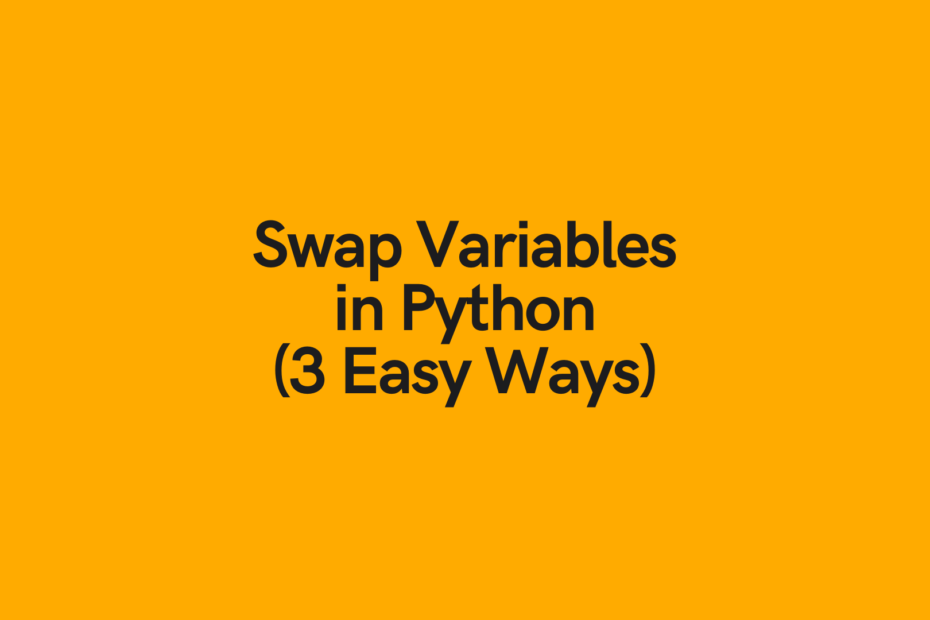# 3 Ways to Swap Variables in PythonIn this tutorial, you’ll learn how to swap variables in Python, using temporary and without temporary variables. Being able to swap variables can be a useful skill to learn and has many practical applications such as in game development and web development. You’ll learn three different ways to swap variables in Python, including using temporary variables, without using temporary variables, and using arithmetic operations.

The Quick Answer: Swap Variables in Python with Tuple Unpacking

## Swap Variables in Python without a Temporary Variable

The most “pythonic” way to swap variables in Python is without a temporary variable. This method is also often referred to as tuple swapping.

Before explaining how this method works, let’s take a look at what it looks like:

``````# Swap Variables in Python without a Temporary Variable
x = 3
y = 6

x, y = y, x

print('x equals: ', x)
print('y equals: ', y)

# Returns:
# x equals:  6
# y equals:  3``````

We can see that this is a very easy and, importantly, readable way of swapping variables in Python. In fact, it’s likely the most Pythonic way to swap variables, given how understandable it is.

But how does this work? Is there some under the hood magic that allows us to do this? Not really. When we assign our variables to two values separated by commas, they return a tuple.

In Python, when we create an evaluative expression with the `=` sign, the right side of the expression is evaluated first. Because of this, when we assign something to ` = y, x`, this is evaluated first. Behind the scenes, Python actually creates a tuple containing the two objects.

Let’s confirm this by creating the tuple and printing the type:

``````# Checking how tuples are created
x = 3
y = 6
z = x, y

print(z)
print(type(z))``````

We can see that by separating values by a comma on the right side of the equal sign, that a tuple is created. Then, when we assign multiple values on the right, we implicitly unpack the values in the tuple.

Since the left-hand side of the expression is composed of two identifiers, the tuple is unpacked in the order that they appear in the tuple. Since the items appear in the reverse order on the right side compared to on the left side, the variables are effectively switched or swapped, implicitly. This is a highly readable method of doing things, as we’re able to understand it quite intuitively.

Now that we know this, let’s see what the operation looks like:

``````# Understanding variable swapping without temporary variables in Python
x = 3
y = 6

x, y = y, x
# Is the same as: x, y = (y, x)

print('x equals: ', x)
print('y equals: ', y)

# Returns:
# x equals:  6
# y equals:  3``````

We can see from the code above that our implicit tuple would return the same as an explicit tuple.

In the next section, you’ll learn how to swap variables in Python using a temporary variable.

## Swap Variables in Python with a Temporary Variable

In this section, you’ll learn how to use a temporary variable. This method is equally valid, but requires the declaration of a third variable. Since the third variable isn’t used following the swapping of variables, the method explained above is preferred. That being said, it’s important to understand the different methods at your disposal.

Let’s see what this method looks like in Python:

``````# Swap Variables in Python with a Temporary Variable
x = 3
y = 7

z = x
x = y
y = z

print('x equals: ', x)
print('y equals: ', y)

# Returns:
# x equals:  7
# y equals:  3``````

This example, while valid is a bit more confusing. We first assign the value of one variable to our third variable. Then we assign the first to the second variable, and the second to the third. (Even writing this was confusing, so I don’t blame you if you want to forget this method altogether and stick to the first method).

The reason this works is immediately understandable. However, it is difficult to understand and not the most intuitive. Because of this it can often be better simply to stick to the first method.

## Swap Variables in Python Using Arithmetic

In this final section, you’ll learn a third method to swap Python variables for numeric values. We’ll be using arithmetic expressions to swap our variables – because of this it’ll only work with numerical values.

Let’s see what this approach looks like and later dive into why this approach works:

``````# Swap Variables with Arithmetic
x = 3
y = 4

x = x + y       # x = 7, y = 4
y = x - y       # x = 7, y = 3
x = x - y       # x = 4, y = 3

print('x equals: ', x)
print('y equals: ', y)

# Returns:
# x equals:  4
# y equals:  3``````

This method works because we’re able to work with the interplay of the numbers. Again, it’s not the most practical approach, since it does require three lines where a single line would do. However, it is a good exercise to understand and to keep in your back pocket for coding interviews.